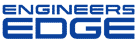# Re: True position calculation

[ Home ][ Forum Archive ] [ Active Engineering Forum ]

Posted By<" ">Jeff M on May 23, 2002 at 14:51:19:

In Reply to: True position calculation posted byTerri O'Day on May 23, 2002 at 14:43:07:

: Can someone explain why their is a factor of 2 in the calculation for true position ?
: 2 *SqRT(delta X ^2)+ (delta y^2).

true position is a circle surrounding the theoretical center location of a feature. If the feature is off from that theoretical center by "x" and "y", then you need to calculate the true position to assure that it lies within the specified tolerance zone. If the x and y dimension is known, then you can calculate the distance from the theoretical center using the pythagorean theorm, which is sqrt(x^2 + y^2). That value is then doubled (X 2) to get your circular projected tolerance zone around the theoretical center. Hope that is clear!?!?

< "> Subject: Re: Re: True position calculation